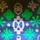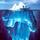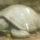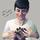## General Question# A Chemistry problem for those chemist masterminds out there?

Asked by 2CDenzy (442) May 31st, 2011

This is on a study guide for a chemistry exam, I understand all the terms and how they can apply but this seems complicated to me.

An aqueous solution containing 288 g of a nonvolatile compound having the stoichiometric composition C(n)H(2n)O(n) in 90.0 g of water boils at 101.24*C at 1.00 atm pressure. What is the molecular formula of the compound?

The answer is C(45)H(90)O(45) but I don’t understand everything it takes to get there. If anyone could help me out it would be much appreciated.

Observing members: 0Composing members: 0I studied chemistry long ago, but I know the molecular weight of water is 18, so 90 g of water is 5 moles. One mole of C(n)H(2n)O(n) weighs 12n + 2n + 16n = 30n grams.

Normally this isn’t enough information, but from the boiling point elevation of water (given that the solution is aqueous) you can calculate the molality of the solution—here’s where I’d have to crack some books. Then you infer how many moles M of solute (weighing 288 grams) are in solution, from which n = 288*M / 30 = 9.6 * M.

If your answer is correct (n=45) then M must work out to 4.68 moles.

gasman (11315)“Great Answer” (1) Flag as…I think @gasman is spot on but I also forget a lot of the details. If you get the solution, please post the method. I’d be nice to get the review of chemistry.

roundsquare (5517)“Great Answer” (0) Flag as…Fluther mods, I know I’m not allowed to give direct answers to homework questions, but as this student appears to just be doing practice problems is it okay? Sorry if I’m misbehaving here!
Let me think; it’s been a few years.

The pertinent equation is ΔT = Kb · mb · i, meaning change in boiling point (the difference between the elevated boiling point and the regular boiling point for that solvent) is equal to the constant Kb (for water it is .512), times mb (the molality of the solution) times i (the van’t Hoff factor, which is a fancy name for a whole number multiple that you have to include if the molecules of solute dissolve into separate pieces. An organic molecule like this won’t dissolve, though, so i = 1 and you don’t really need to include it).

So your ΔT is 1.24 (as water normally boils at 100 degrees C) and your Kb is .512. Now you can solve for the molality, which comes out to be 2.42. Molality is defined as being moles of solute over mass of solvent. Careful, the molality formula uses kilograms as the unit on the mass of the solvent. You know the mass of your solvent is 90g, or .090kg, so you can solve for number of moles of solute. I got .218.

Now you know that .218 moles of your mystery molecule have a mass of 288g. Divide by .218: 1 mole of your molecule would have a mass of 1320g (organic molecules can be very heavy!). Using the atomic masses of carbon, hydrogen, and oxygen, you can set up the clever little formula @gasman did. One mole of C(n)H(2n)O(n) weighs 12n + 2n + 16n or 30n. Do you see where that came from? But you also know that one mole eqals 1320g. Soooo:
30n = 1320g
n =...... well I got 44. But I did this ultra quickly and probably did some really sloppy rounding. Why don’t you go through, try and do it neater than I did, find where I screwed up, and also make sure you understand the process I used. Notice I didn’t bother using units throughout the problem too, which is a big no no – it could easily lead to you screwing up something like forgetting that you need to use kilos in that one spot (which I did initially), so just don’t do it!

Mariah (25876)“Great Answer” (5) Flag as…or